# How to calculate total hours worked in a week in Microsoft Excel

In this tutorial, we will learn how to calculate the total hours worked in Microsoft Excel. There are several ways to compute the total hours worked in Microsoft Excel. For this, we will first determine the total working hours for each day and then add up these daily hours for the week using the SUM function.

Currently, we have a data set showing the weekly working schedule of an employee. The Start time and the End time for each day of the week are listed. First, we will calculate the daily working hours and then calculate the total working hours using the SUM function.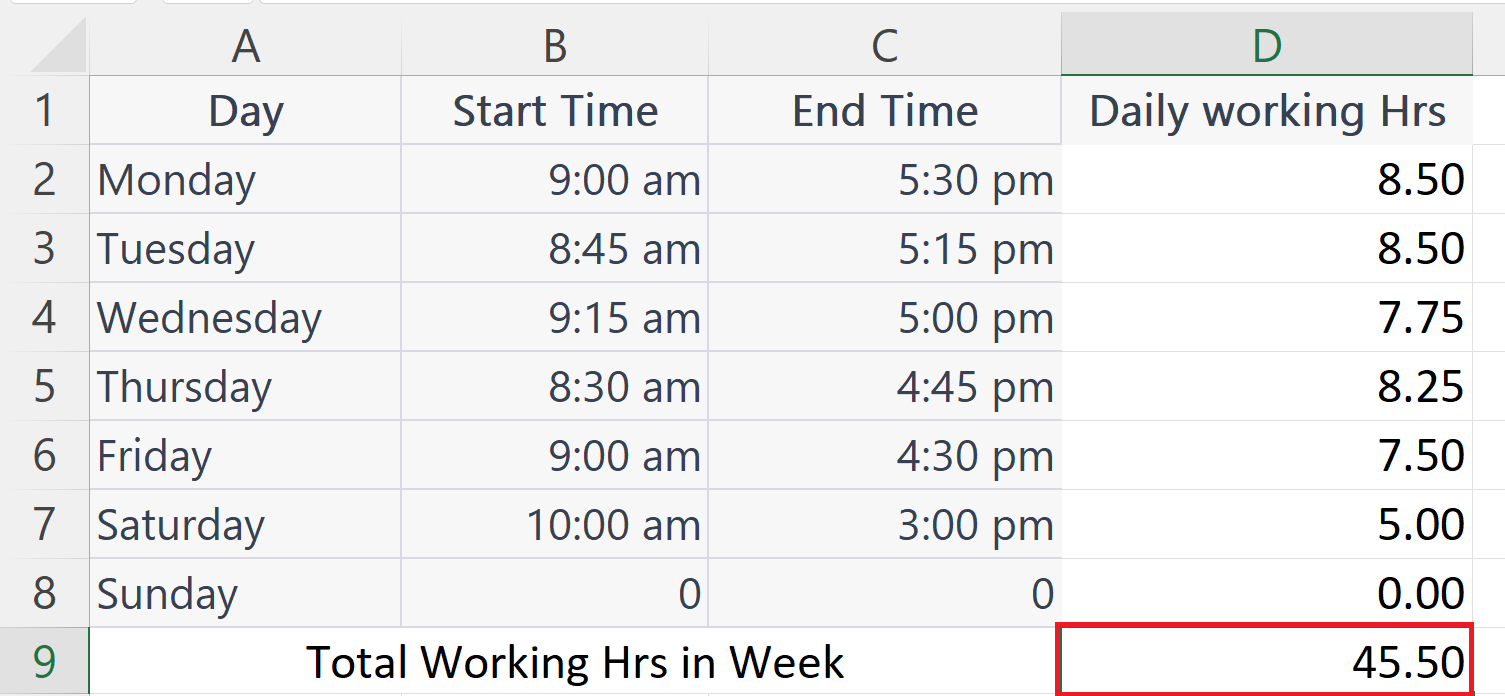Calculating the total working hours in Excel is an essential task in Microsoft Excel. It helps individuals and businesses to monitor and track their work hours and improve their productivity. By accurately recording and tracking the hours worked, employees can assess their efficiency and identify opportunities to increase their productivity. Also, calculating total working hours is crucial for accurately calculating and processing payroll.

### Step 1 – Select a Blank Cell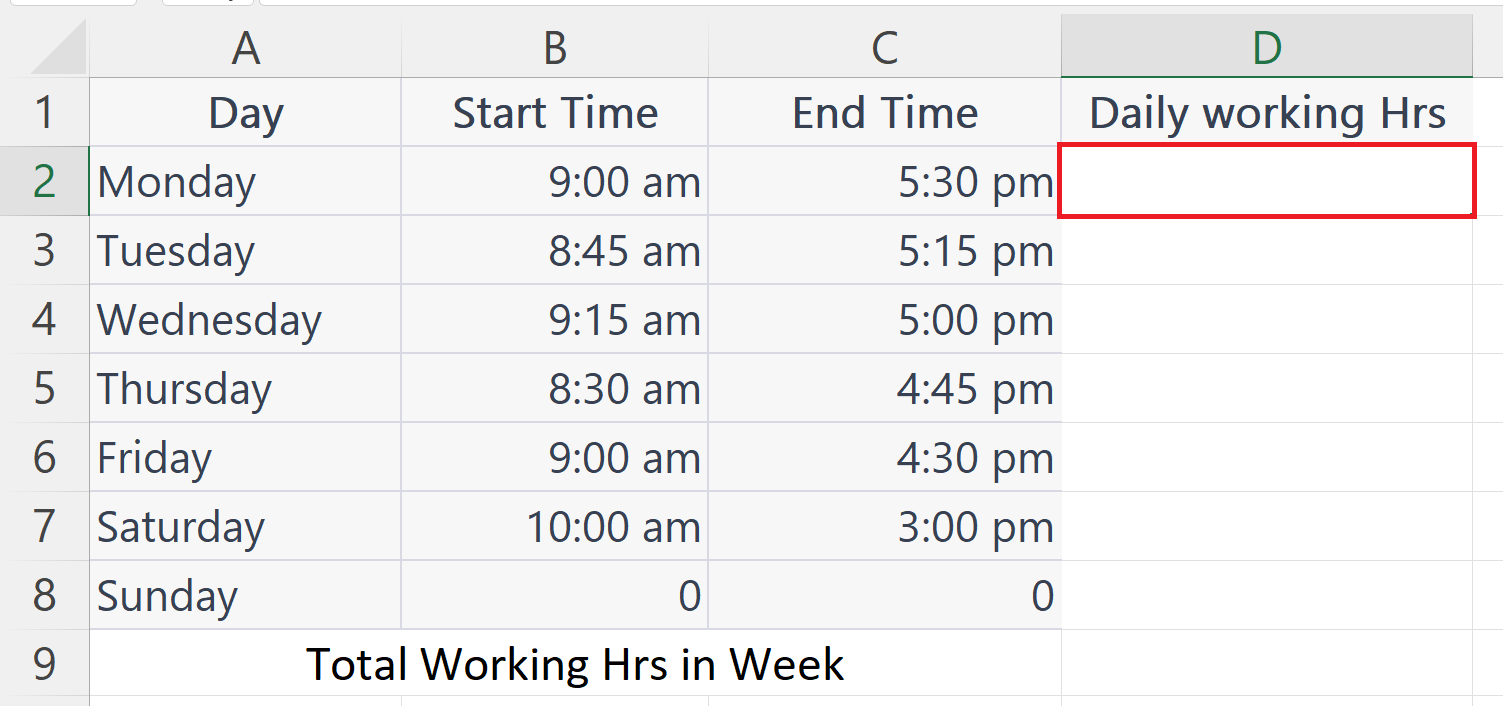– Select a blank cell to calculate the daily working hours.

### Step 2 – Place an Equals sign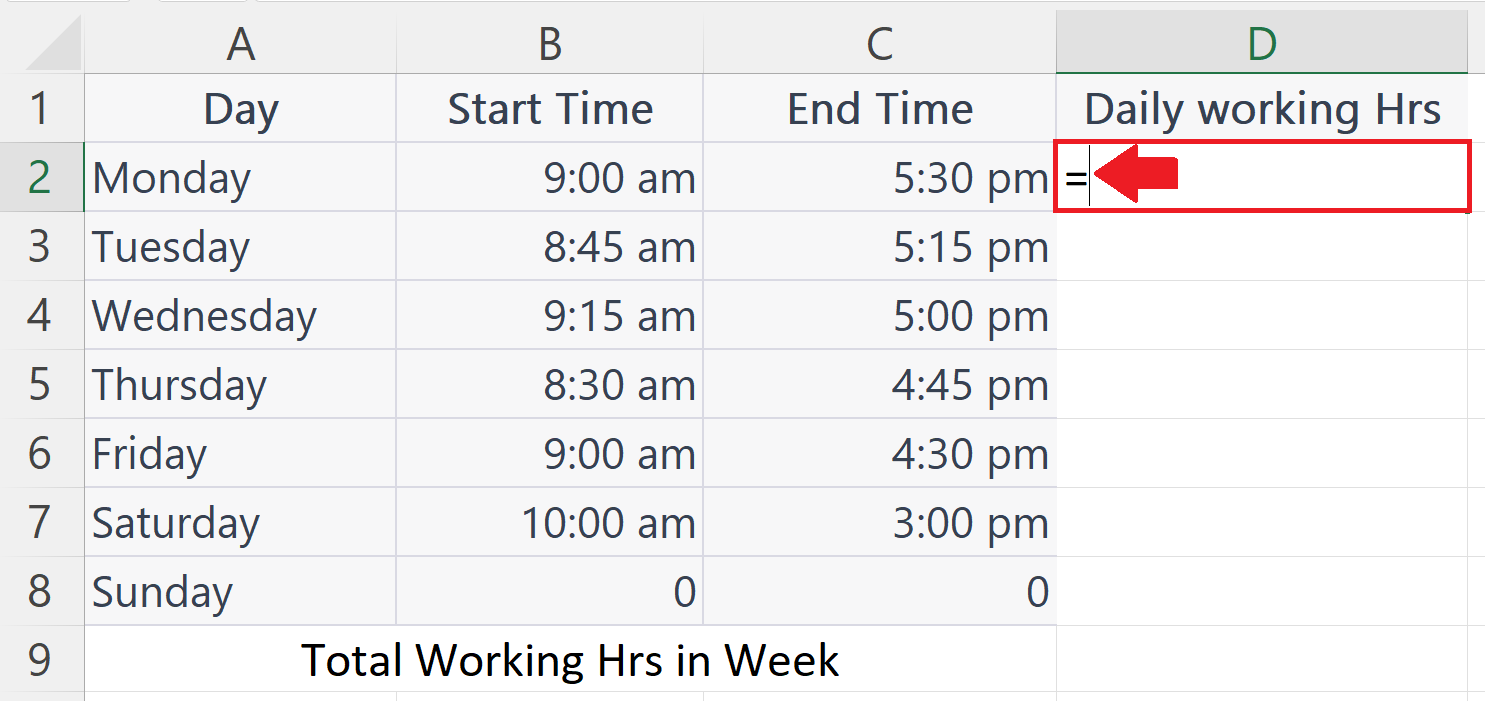### Step 3 – Subtract the Start Time from the End Time– Open a parenthesis and subtract the Start time from the End time.
– Close the Parenthesis.

### Step 4 – Press the Enter Key– Press the Enter key.

### Step 5 – Change the Cell Format to Number Format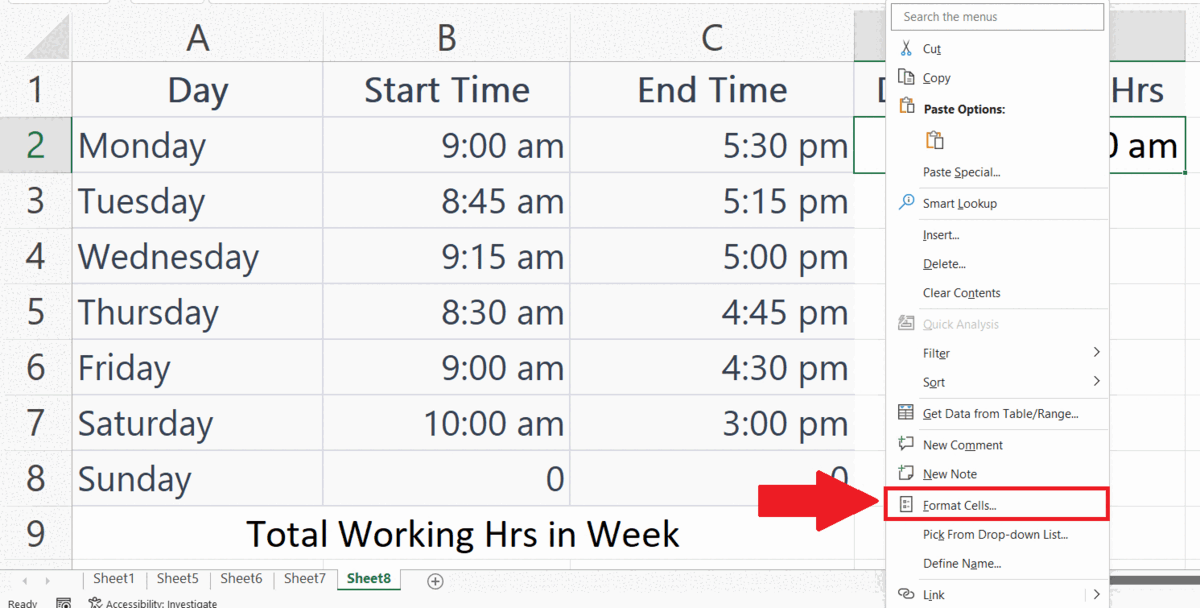– Right-click on the cell.
– Go to the Format Cells Option.
– Select the Number format and Click on OK in the – Format cells dialogue box.

### Step 6 – Multiple the Value with 24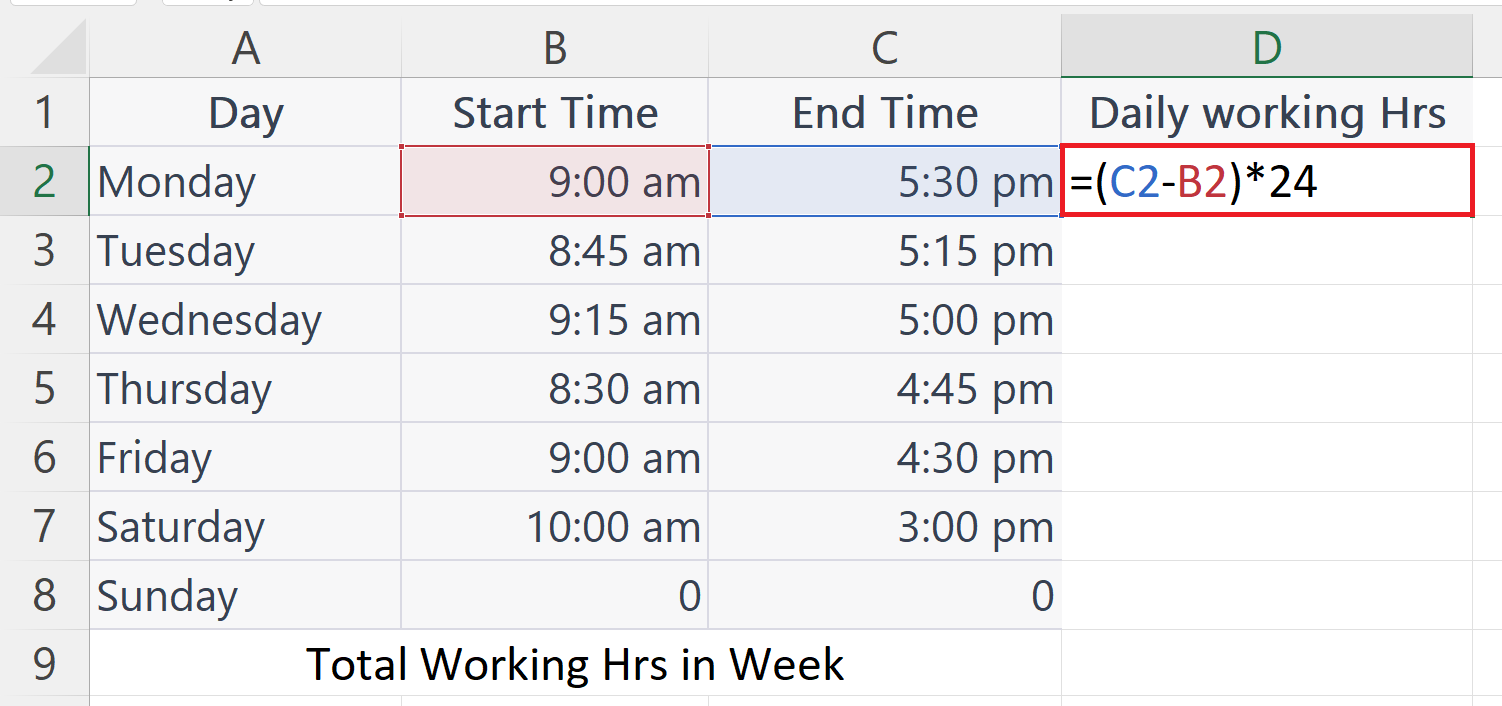– Now multiply the value in the cell by 24.

### Step 7 – Press the Enter Key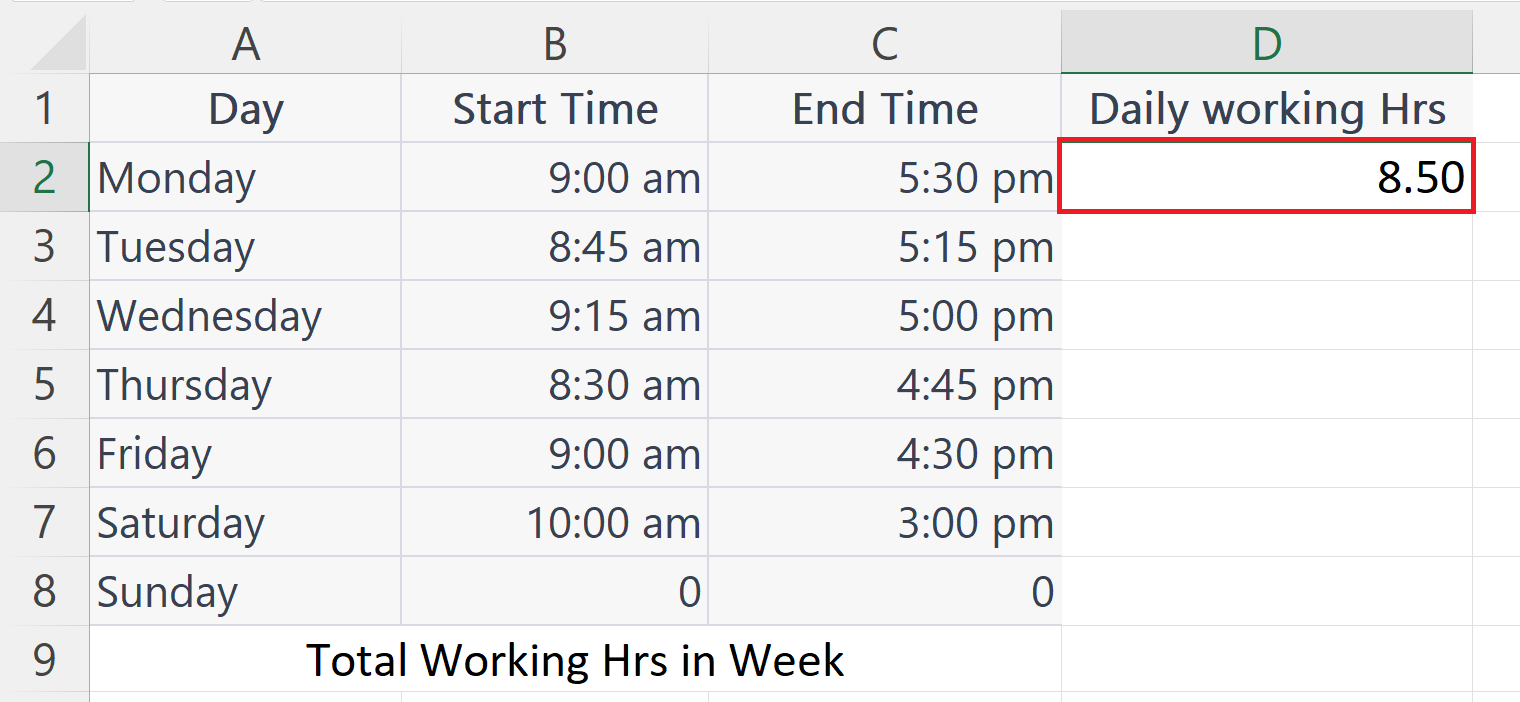– Press the Enter key.
– The working hours for the day will be displayed in the cell.

### Step 8 – Calculate Working Hours for Each Day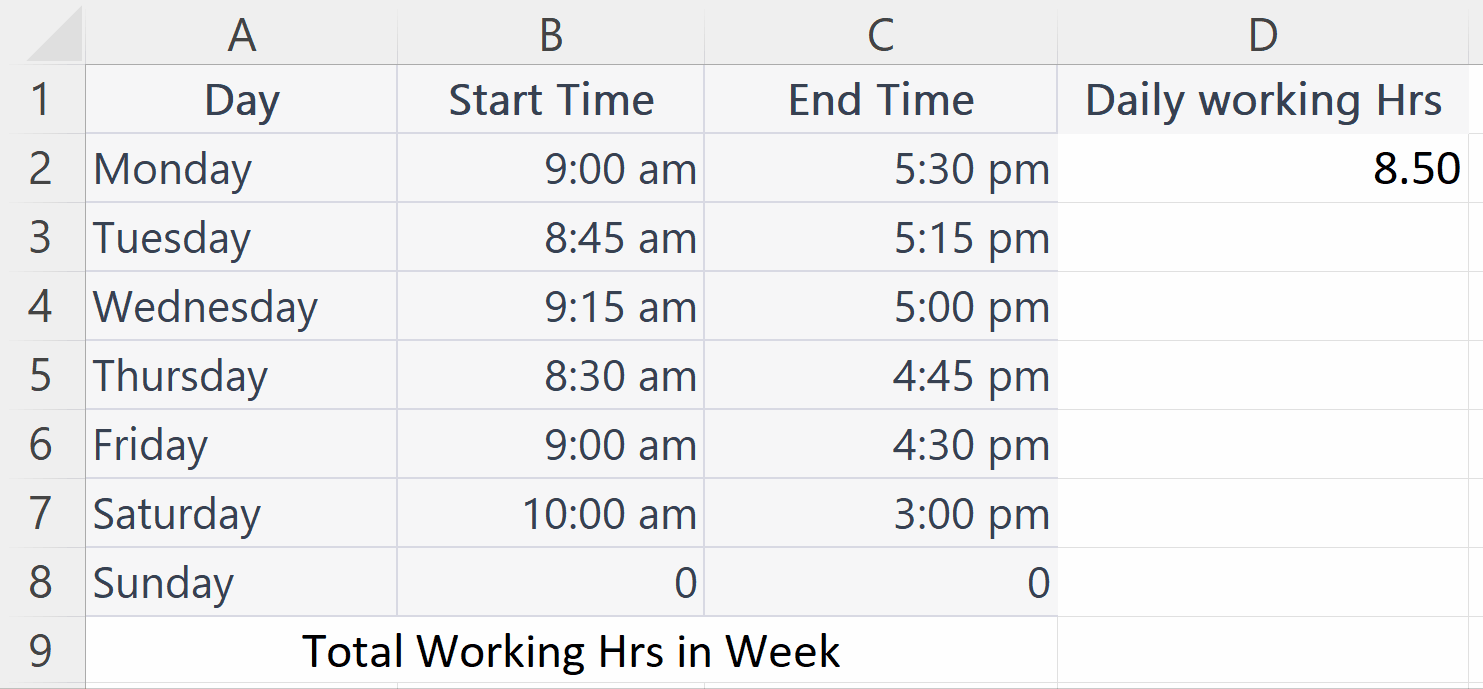– Calculate the working hours for each day using the Auto Fill feature as shown above.

### Step 9 – Select a blank Cell– Select a Blank cell where you want to calculate the total working hours in the week.

### Step 10 – Use the SUM function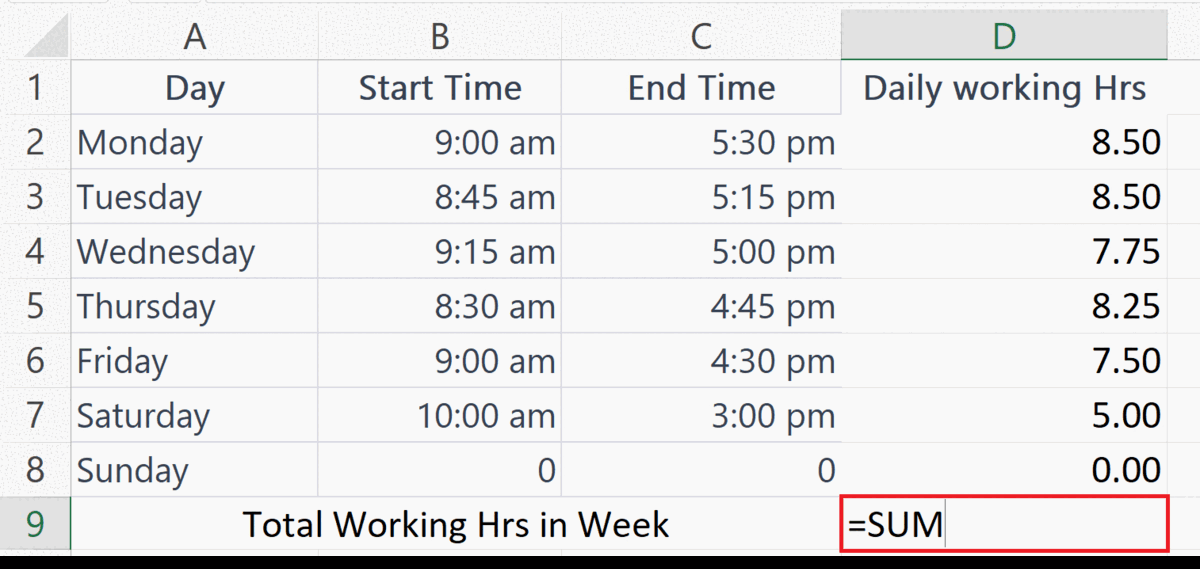– Place an Equals sign and enter the SUM function.
– Add the cell range containing the working hours for each day in the SUM function.

### Step 11 – Press the Enter Key– Press the Enter key.
– The total working hours for a week will be displayed in the cell.